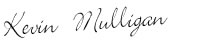# How to Figure Rate of Return on Investments

June 5, 2013We don’t invest money in our retirement accounts just to see what happens. We all want high returns, positive growth, and a big pile of cash to retire on. Investing your funds through automatic investments is a great way to start, but at some point you have to do some math to figure out if you are earning a return or not.

This is surprisingly difficult.

Brokerage firms and mutual fund companies don’t want to make it easy for you to calculate your personal rate of return on an investment. Why? Because you might be able to see that the marketing materials they put out aren’t exactly accurate.

Inaccurate marketing about the rate of return on mutual funds is somewhat inevitable even if the companies wanted to give you accurate information. This is because every investor will invest money into the fund at different times, at different amounts, and at different intervals. Unless all investors are following the exact same investment plan then the returns will be different.

That’s why knowing how to calculate your own rate of return on your investments is so important. Don’t accept simple marketing material or vague reports at your return. Here’s how to calculate your rate of return.

## How to Calculate Accurate Rates of Return on Your Investments

There are three methods to calculate your returns. You should use two of them at best.

### Do Not Use Arithmetic Rate of Return

Using average annual rate of return or average annual arithmetic return will not be an accurate showing of your rate of return on your investments. It is a simple equation where you add up all of the returns for the time periods you are looking at, then divide by the number of time periods.

The average annual rate of return can be calculated as: (Rate of Return Year 1 + Rate of Return Year 2 + Rate of Return Year N) / N.

For example, you invest \$1,000 (Investment) and in one year it grows to \$1,500. Your return is \$500. (Return Year 1). The following year it drops back to \$1,000 (Return Year 2) and you return is -\$500.

The first year’s rate of return is 50% (\$500 growth / \$1,000 investment). The second year’s rate of return is -33.33% (-\$500 growth, divided by \$1,500 investment).

If you add them together and divide by two years, you get 8.5% as the average annual rate of return. (50% minus 33% = 17%; 17% divided by 2.)

The average annual rate of return over the two years is positive and quite healthy at 8.5%. However the actual return is 0% because the money grew to \$1,500 then shrank back to the starting investment amount of \$1,000. We can also tell this is inaccurate because if you multiply \$1,000 by 8.5% growth (and then again by 8.5% growth) you would end up with \$1,177.23 instead of \$1,000.

### Use Geometric Rate of Return

A far better calculation is compound rate of return, also known as average annual geometric return or time-weighted rate of return. This shows how much the investment grew due to compounding over a certain number of time periods.

Hang with me â€“ incoming math!

To use the time-weighted rate of return we need:

1. The rate of return for each time period (50% in year 1 and -33% using our example above)
2. Add one to each rate of return (50% becomes 1.50 and -33% becomes .667)
3. Multiply the rates of return together from step 2 (1.50 x .667 = 1.0005)
4. Take the root of the product in step 3. The root number is equal to the number of time periods. In our example the number of periods is 2, so we need the 2nd root of the product in step 3. (2nd root of 1.0005 = 1.00025)
5. Subtract one from the result (0.00025; 0% rate of return due to rounding)

However, there is only one problem with geometric mean rate of return . . . it only tells us what the rate of return is on one chunk of money invested over time. It doesn’t take into account (or at least, not very easily) dividends and other contributions to your retirement accounts.

### Use XIRR or Internal Rate of Return

Enter the XIRR formula to tell us how our portfolio grew. XIRR is a spreadsheet formula that is used to account for cash flows.

To use it, you need:

• a spreadsheet program like Excel, OpenOffice, or LibreOffice (the last two are free open-source tools)
• dates and amounts you contributed to your portfolio
• beginning and ending balance of your portfolio

The spreadsheet does the rest. Of course if you are using dollar cost averaging and investing every week or two weeks . . . and have been doing this for years . . . then you’ve got some data entry in your future.

Million Dollar Journey has an excellent post explaining how to use XIRR to calculate your portfolio’s returnsÂ that includes a simple example.

## How Should I Calculate My Portfolio’s Returns?

At the end of the day, even though it is more complicated to use, XIRR is going to tell you exactly the annualized rate of return you received on your investments. If you had a lump sum of cash that you invested, never received dividends on, and never contributed to again then you could use geometric mean. Otherwise XIRR is going to be the most accurate.Kevin Mulligan is a debt reduction champion with a passion for teaching people how to budget and stay out of debt. He's building a personal finance freelance writing career and has written for RothIRA.com, Discover Bank, ING Direct, and many others.

All posts by

•Thomas | Your Daily Finance